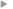## Numerical discretization causing error variance loss and the need for inflation

#### Ménard, Richard ; Skachko, Sergey ; Pannekoucke, Olivier

Année de publication
2021
Résumé
<p align=justify>The effects of model discretization errors on the propagation of error covariance have a more complex nature than the effect on the state variable. The analysis is carried out for the advection transport equation, where the continuous (in space and time) propagation of the related error covariance function can be written, solved and compared with the discrete model applied to the covariance matrix. The numerical analysis of the problem is carried out with a 1D-problem, but is also illustrated with a 3D chemical transport model (CTM) used for chemical data assimilation. It is shown that variance loss (compared to the continuous propagation solution) depends on the covariance function itself as well as the numerical discretization scheme. The variance loss is particularly sensitive to the correlation length. In a simple first-order discretization, an analytical expression is obtained and is used to derive an analytical expression for inflation. Experiments show, for example, that following an analysis over a dense network of observations (with spatially uncorrelated errors) a significant variance loss occurs in the propagation step. With the (variance) inflation scheme, we are able to restore the variance lost at each grid point, and at each timestep, during the entire integration. The variance inflation scheme applied to an EnKF can be formulated to change the variance spread of the ensemble or to act directly on the state.</p>
Texte intégralAccès à la notice sur le site du portail documentaire de Météo-FranceListe complète des notices publiques